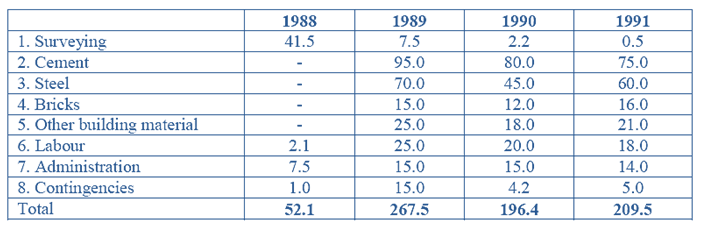## Sunday, 2 April 2017

### DI QUIZ:BASED ON NEW PATTERN

Direction for the questions: The table below shows the estimated cost (in Rs. Lakh) of a project of laying a railway line between two places.Question 1: The total expenditure is required to be kept within Rs. 700 lakh by cutting the expenditure on administration equally in all the years. What will be the percentage cut for 1989?
(a) 22.6
(b) 32.6
(c) 42.5
(d) 52.6

Question 2: If the length of line to be laid each year is in proportion to the estimated cost for material and labour, what fraction of the total length is proposed to be completed by the third year?
(a) 0.9
(b) 0.7
(c) 0.6
(d) 0.3
Question 3: What is the approximate ratio of the total cost of materials for all the years to the total labour cost?
(a) 4 : 1
(b) 8 : 1
(c) 12:1
(d) 16 : 1
Question 4: If the cost of materialsrise by 5% each year from 1990 onwards, by how much will the estimated cost rise?
(a) Rs. 11.4 lakh
(b) Rs. 16.4 lakh
(c) Rs.21.4 lakh
(d) Rs.26.4 lakh
Question 5: It is found at the end of 1990, that the entire amount estimated for the project has been spent. If for 1991, the actual amount spent was equal to that which was estimated, by what percent (approximately) has the actual expenditure exceeded the estimated expenditure?
(a) 39
(b) 29
(c) 19
(d) 9

Step-1 to solve this question is to calculate the total expenditure = 52.1+267.5+196.4+209.5 = 725.5 lakhs.
Now the question states that the expenditure has to be limited to/kept within 700 lakhs.
This means that the total expenditure has tobe reduced by 25.5 lakhs.
Thus, each year, the expenditure has to reduce by = (25.5/4) = 6.375 lakhs.
Therefore, percentage reduce for administration in 1989 would be = (6.375/15) × 100 = 42.5%.
Let’s use list down the costs of material and labor( in lakhs) for the individual years:
Cost for 1988 = 2.1
Cost for 1989 = 95+70+15+25+25 = 230
Cost for 1990 = 80+45+12+18+20 = 175
Cost for 1991 = 75+60+16+21+18 = 190
Thus, proportion of these expenditures till 1990 = (2.1 + 230 + 175) / (2.1 + 230 + 175 + 190) = 0.6817. Remember, this also will be be the fraction of the total length of the line.
The total material cost is (using values from the given table) = (95+80+75+70+45+60+15+12+16+25+18+21) = 532
The total labour cost is (using values from the given table) = (2.1+25+20+18) = 65.1
Now, calculating the ration: = 532 : 65.1
This is approximately = 8 : 1